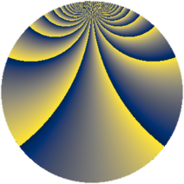# Properties

 Label 672.4.clLevel $672$ Weight $4$ Character orbit 672.cl Rep. character $\chi_{672}(5,\cdot)$ Character field $\Q(\zeta_{24})$ Dimension $3040$ Sturm bound $512$

# Related objects

## Defining parameters

 Level: $$N$$ $$=$$ $$672 = 2^{5} \cdot 3 \cdot 7$$ Weight: $$k$$ $$=$$ $$4$$ Character orbit: $$[\chi]$$ $$=$$ 672.cl (of order $$24$$ and degree $$8$$) Character conductor: $$\operatorname{cond}(\chi)$$ $$=$$ $$672$$ Character field: $$\Q(\zeta_{24})$$ Sturm bound: $$512$$

## Dimensions

The following table gives the dimensions of various subspaces of $$M_{4}(672, [\chi])$$.

Total New Old
Modular forms 3104 3104 0
Cusp forms 3040 3040 0
Eisenstein series 64 64 0

## Trace form

 $$3040q - 12q^{3} - 8q^{4} - 16q^{7} - 4q^{9} + O(q^{10})$$ $$3040q - 12q^{3} - 8q^{4} - 16q^{7} - 4q^{9} - 24q^{10} - 12q^{12} - 488q^{16} - 4q^{18} - 24q^{19} - 8q^{21} - 32q^{22} - 12q^{24} - 8q^{25} - 16q^{28} - 1196q^{30} - 48q^{31} - 24q^{33} + 864q^{36} - 8q^{37} - 4q^{39} - 24q^{40} - 908q^{42} - 32q^{43} - 12q^{45} - 8q^{46} - 220q^{51} - 4992q^{52} - 12q^{54} - 16q^{57} - 2024q^{58} + 252q^{60} - 24q^{61} - 16q^{63} - 6080q^{64} - 12q^{66} + 3256q^{67} - 16q^{70} - 4q^{72} - 24q^{73} - 1512q^{75} - 11720q^{78} + 10416q^{82} + 2404q^{84} - 2032q^{85} - 12q^{87} - 8q^{88} - 3616q^{91} - 220q^{93} - 14064q^{94} - 19392q^{96} - 16q^{99} + O(q^{100})$$

## Decomposition of $$S_{4}^{\mathrm{new}}(672, [\chi])$$ into newform subspaces

The newforms in this space have not yet been added to the LMFDB.